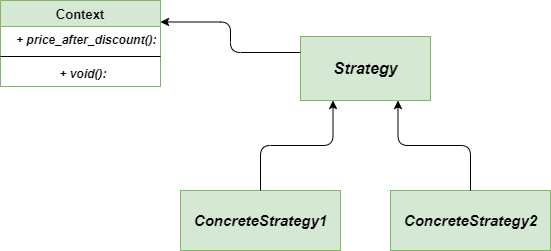Open in App
Not now

# Strategy Method – Python Design Patterns

• Last Updated : 15 Dec, 2021

The strategy method is Behavioral Design pattern that allows you to define the complete family of algorithms, encapsulates each one and putting each of them into separate classes and also allows to interchange there objects. It is implemented in Python by dynamically replacing the content of a method defined inside a class with the contents of functions defined outside of the class. It enables selecting the algorithm at run-time. This method is also called as Policy Method.

### Problem without using Strategy Method

Imagine you created an application for the departmental store. Looks simple? Initially, there was one and only one type of discount called the On-Sale-Discount. So. everything was going with ease and there was no difficulty for maintaining such a simple application for a developer but as time passes, the owner of the departmental store demands for including some other types of discount also for the customers. It is very easy to say to make these changes but definitely not very easy to implement these changes in an efficient way.

### Solution using Strategy method

Let’s see how can we solve the above-described problem in an efficient way. We can create a specific class that will extract all the algorithms into separate classes called Strategy. Out actual class should store the reference to one of the strategy class.

## Python3

 `"""A separate class for Item"""``class` `Item:` `    ``"""Constructor function with price and discount"""` `    ``def` `__init__(``self``, price, discount_strategy ``=` `None``):``        ` `        ``"""take price and discount strategy"""``        ` `        ``self``.price ``=` `price``        ``self``.discount_strategy ``=` `discount_strategy``        ` `    ``"""A separate function for price after discount"""` `    ``def` `price_after_discount(``self``):``        ` `        ``if` `self``.discount_strategy:``            ``discount ``=` `self``.discount_strategy(``self``)``        ``else``:``            ``discount ``=` `0``            ` `        ``return` `self``.price ``-` `discount` `    ``def` `__repr__(``self``):``        ` `        ``statement ``=` `"Price: {}, price after discount: {}"``        ``return` `statement.``format``(``self``.price, ``self``.price_after_discount())` `"""function dedicated to On Sale Discount"""``def` `on_sale_discount(order):``    ` `    ``return` `order.price ``*` `0.25` `+` `20` `"""function dedicated to 20 % discount"""``def` `twenty_percent_discount(order):``    ` `    ``return` `order.price ``*` `0.20` `"""main function"""``if` `__name__ ``=``=` `"__main__"``:` `    ``print``(Item(``20000``))``    ` `    ``"""with discount strategy as 20 % discount"""``    ``print``(Item(``20000``, discount_strategy ``=` `twenty_percent_discount))` `    ``"""with discount strategy as On Sale Discount"""``    ``print``(Item(``20000``, discount_strategy ``=` `on_sale_discount))`

Output:

```Price: 20000, price after discount: 20000
Price: 20000, price after discount: 16000.0
Price: 20000, price after discount: 14980.0 ```

### Class Diagram

Following is the class diagram for the Strategy Methodclass-diagram-Strategy-method

• Open/Closed principle: Its always easy to introduce the new strategies without changing the client’s code.
• Isolation: We can isolate the specific implementation details of the algorithms from the client’s code.
• Encapsulation: Data structures used for implementing the algorithm are completely encapsulated in Strategy classes. Therefore, the implementation of an algorithm can be changed without affecting the Context class
• Run-time Switching: It is possible that application can switch the strategies at the run-time.

• Creating Extra Objects: In most cases, the application configures the Context with the required Strategy object. Therefore, the application needs to create and maintain two objects in place of one.
• Awareness among clients: Difference between the strategies should be clear among the clients to able to select a best one for them.
• Increases the complexity: when we have only a few number of algorithms to implement, then its waste of resources to implement the Strategy method.

### Applicability

• Lot of Similar Classes: This method is highly preferred when we have a lot of similar classes that differs in the way they execute.
• Conquering Isolation: It is generally used to isolate the business logic of the class from the algorithmic implementation.

Further Read – Strategy Method in Java

My Personal Notes arrow_drop_up